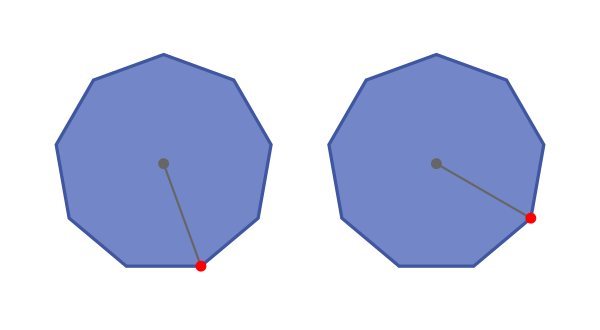# Nonagons - polygons with 9 sides

By Martin McBride, 2022-10-09
Tags: nonagon 9 sided shape regular polygon irregular polygon
Categories: gcse geometryA nonagon is a flat shape with 9 straight sides.

## Regular and irregular nonagons

A regular nonagon is a 9-sided shape where every side is the same length and every corner has the same angle. All regular nonagons have the same shape, like this: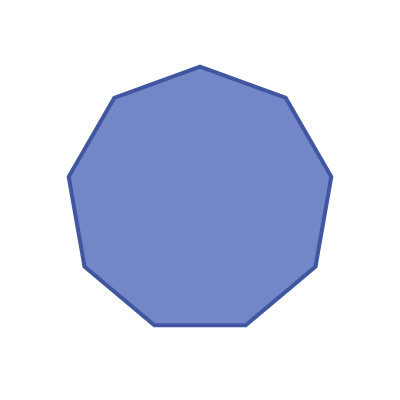An irregular nonagon is a 9 sides shape where not every side and angle are equal. There are many different irregular nonagon shapes, here is an example: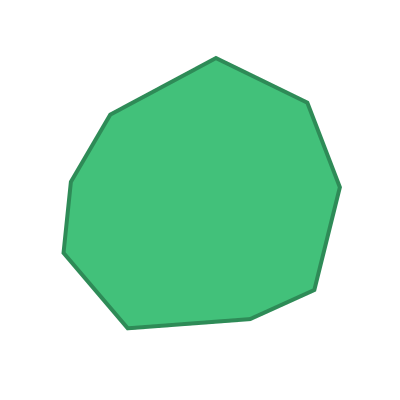## Name

The name nonagon is a combination of the words nonus (Latin meaning ninth) and gonon (Latin meaning angle or corner).

This is slightly unusual because most polygons use greek numbers in their name. An alternative name for a nonagon is enneagon, based on the Greek word ennea (meaning nine). This name isn't used very often.

Nonagons are sometimes called 9-gons.

## Interior angles

The interior angles of a nonagon are shown here: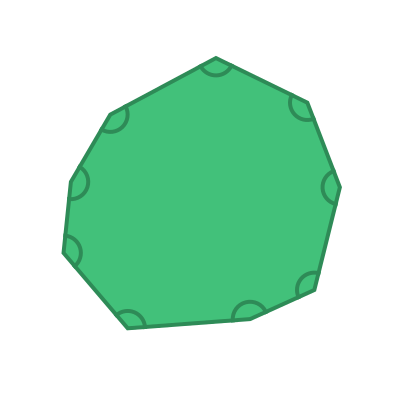The sum of these 9 angles is given by the formula:

sum of interior angles = (n - 2) x 180


Where n is the number of sides. In this case, the number of sides n is 9, so the sum of the interior angles is:

(9 - 2) x 180 = 1260 degrees


For a regular nonagon, all the interior angles are equal: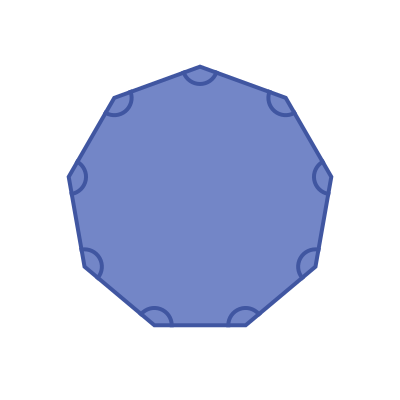This means that the interior angle of a regular nonagon is:

1260 / 9 =  140 degrees


## Exterior angles

The exterior angles of a nonagon are shown here: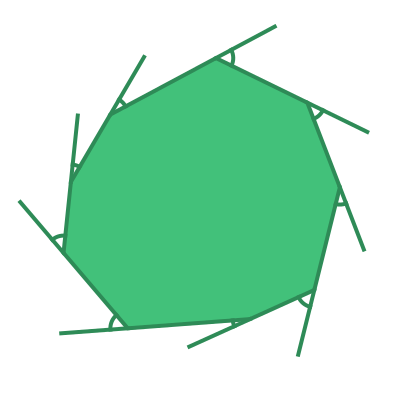The sum of the exterior angles of any polygon is 360 degrees.

For a regular nonagon, all the interior angles are equal: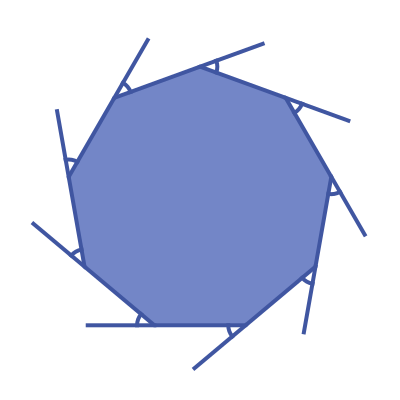This means that the exterior angle of a regular nonagon is:

360 / 9 =  40 degrees


## Symmetry of regular nonagons

A regular nonagon has 9 lines of symmetry. This means that it can be reflected over each of the 9 grey lines shown here: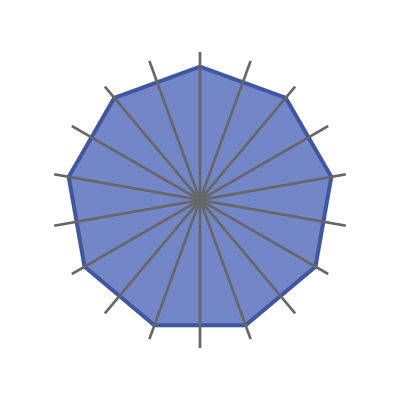A regular nonagon has rotational symmetry of order 9. This means that if it rotated about its centre by a 9th of a full turn, it will map onto itself: